# High School Physics : Using Ohm's Law

## Example Questions

2 Next →

### Example Question #11 : Using Ohm's Law

Ohm's Law describes the relationship between which variables?

Electric field, current, power

Voltage, electric field, power

Voltage, current, resistance

Resistance, power, electromotive force

Power, resistivity, electromotive force

Voltage, current, resistance

Explanation:

Ohm's law can be written as: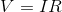It demonstrates that voltage is equal to the product of current and resistance within an electric circuit.

### Example Question #12 : Electricity And Magnetism

The current in a circuit is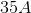and the voltage is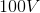. What is the resistance?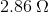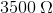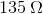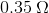Explanation:

Ohm's law says. Plug in our given values to solve.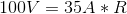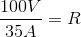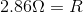2 Next →

### All High School Physics Resources